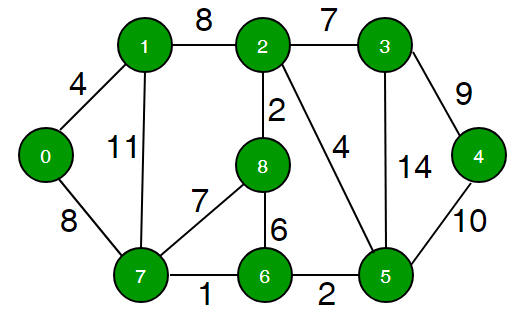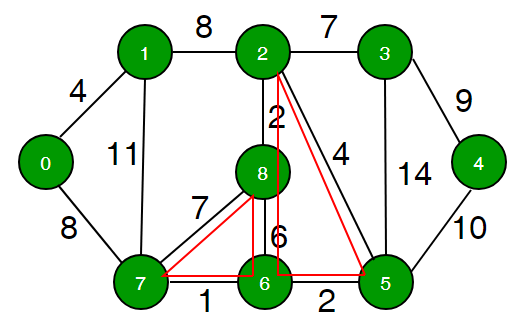Related Articles
Find minimum weight cycle in an undirected graph
• Difficulty Level : Hard
• Last Updated : 22 Dec, 2020

Given a positive weighted undirected graph, find the minimum weight cycle in it.

Examples:`Minimum weighted cycle is :````Minimum weighed cycle : 7 + 1 + 6 = 14 or
2 + 6 + 2 + 4 = 14 ```

The idea is to use shortest path algorithm. We one by one remove every edge from the graph, then we find the shortest path between two corner vertices of it. We add an edge back before we process the next edge.

```1). create an empty vector 'edge' of size 'E'
( E total number of edge). Every element of
this vector is used to store information of
all the edge in graph info

2) Traverse every edge edge[i] one - by - one
a). First remove 'edge[i]' from graph 'G'
b). get current edge vertices which we just
removed from graph
c). Find the shortest path between them
"Using Dijkstra’s shortest path algorithm "
d). To make a cycle we add the weight of the
removed edge to the shortest path.
e). update min_weight_cycle  if needed
3). return minimum weighted cycle```

Below is the implementation of the above idea

## C++

 `// c++ program to find shortest weighted``// cycle in undirected graph``#include``using` `namespace` `std;``# define INF 0x3f3f3f3f``struct` `Edge``{``    ``int` `u;``    ``int` `v;``    ``int` `weight;``};` `// weighted undirected Graph``class` `Graph``{``    ``int` `V ;``    ``list < pair <``int``, ``int` `> >*adj;` `    ``// used to store all edge information``    ``vector < Edge > edge;` `public` `:``    ``Graph( ``int` `V )``    ``{``        ``this``->V = V ;``        ``adj = ``new` `list < pair <``int``, ``int` `> >[V];``    ``}` `    ``void` `addEdge ( ``int` `u, ``int` `v, ``int` `w );``    ``void` `removeEdge( ``int` `u, ``int` `v, ``int` `w );``    ``int` `ShortestPath (``int` `u, ``int` `v );``    ``void` `RemoveEdge( ``int` `u, ``int` `v );``    ``int` `FindMinimumCycle ();` `};` `//function add edge to graph``void` `Graph :: addEdge ( ``int` `u, ``int` `v, ``int` `w )``{``    ``adj[u].push_back( make_pair( v, w ));``    ``adj[v].push_back( make_pair( u, w ));` `    ``// add Edge to edge list``    ``Edge e { u, v, w };``    ``edge.push_back ( e );``}` `// function remove edge from undirected graph``void` `Graph :: removeEdge ( ``int` `u, ``int` `v, ``int` `w )``{``    ``adj[u].``remove``(make_pair( v, w ));``    ``adj[v].``remove``(make_pair(u, w ));``}` `// find the shortest path from source to sink using``// Dijkstra’s shortest path algorithm [ Time complexity``// O(E logV )]``int` `Graph :: ShortestPath ( ``int` `u, ``int` `v )``{``    ``// Create a set to store vertices that are being``    ``// preprocessed``    ``set< pair<``int``, ``int``> > setds;` `    ``// Create a vector for distances and initialize all``    ``// distances as infinite (INF)``    ``vector<``int``> dist(V, INF);` `    ``// Insert source itself in Set and initialize its``    ``// distance as 0.``    ``setds.insert(make_pair(0, u));``    ``dist[u] = 0;` `    ``/* Looping till all shortest distance are finalized``    ``then setds will become empty */``    ``while` `(!setds.empty())``    ``{``        ``// The first vertex in Set is the minimum distance``        ``// vertex, extract it from set.``        ``pair<``int``, ``int``> tmp = *(setds.begin());``        ``setds.erase(setds.begin());` `        ``// vertex label is stored in second of pair (it``        ``// has to be done this way to keep the vertices``        ``// sorted distance (distance must be first item``        ``// in pair)``        ``int` `u = tmp.second;` `        ``// 'i' is used to get all adjacent vertices of``        ``// a vertex``        ``list< pair<``int``, ``int``> >::iterator i;``        ``for` `(i = adj[u].begin(); i != adj[u].end(); ++i)``        ``{``            ``// Get vertex label and weight of current adjacent``            ``// of u.``            ``int` `v = (*i).first;``            ``int` `weight = (*i).second;` `            ``// If there is shorter path to v through u.``            ``if` `(dist[v] > dist[u] + weight)``            ``{``                ``/* If the distance of v is not INF then it must be in``                    ``our set, so removing it and inserting again``                    ``with updated less distance.``                    ``Note : We extract only those vertices from Set``                    ``for which distance is finalized. So for them,``                    ``we would never reach here. */``                ``if` `(dist[v] != INF)``                    ``setds.erase(setds.find(make_pair(dist[v], v)));` `                ``// Updating distance of v``                ``dist[v] = dist[u] + weight;``                ``setds.insert(make_pair(dist[v], v));``            ``}``        ``}``    ``}` `    ``// return shortest path from current source to sink``    ``return` `dist[v] ;``}` `// function return minimum weighted cycle``int` `Graph :: FindMinimumCycle ( )``{``    ``int` `min_cycle = INT_MAX;``    ``int` `E = edge.size();``    ``for` `( ``int` `i = 0 ; i < E ; i++ )``    ``{``        ``// current Edge information``        ``Edge e = edge[i];` `        ``// get current edge vertices which we currently``        ``// remove from graph and then find shortest path``        ``// between these two vertex using Dijkstra’s``        ``// shortest path algorithm .``        ``removeEdge( e.u, e.v, e.weight ) ;` `        ``// minimum distance between these two vertices``        ``int` `distance = ShortestPath( e.u, e.v );` `        ``// to make a cycle we have to add weight of``        ``// currently removed edge if this is the shortest``        ``// cycle then update min_cycle``        ``min_cycle = min( min_cycle, distance + e.weight );` `        ``// add current edge back to the graph``        ``addEdge( e.u, e.v, e.weight );``    ``}` `    ``// return shortest cycle``    ``return` `min_cycle ;``}` `// driver program to test above function``int` `main()``{``    ``int` `V = 9;``    ``Graph g(V);` `    ``// making above shown graph``    ``g.addEdge(0, 1, 4);``    ``g.addEdge(0, 7, 8);``    ``g.addEdge(1, 2, 8);``    ``g.addEdge(1, 7, 11);``    ``g.addEdge(2, 3, 7);``    ``g.addEdge(2, 8, 2);``    ``g.addEdge(2, 5, 4);``    ``g.addEdge(3, 4, 9);``    ``g.addEdge(3, 5, 14);``    ``g.addEdge(4, 5, 10);``    ``g.addEdge(5, 6, 2);``    ``g.addEdge(6, 7, 1);``    ``g.addEdge(6, 8, 6);``    ``g.addEdge(7, 8, 7);` `    ``cout << g.FindMinimumCycle() << endl;``    ``return` `0;``}`

## Python3

 `# Python3 program to find shortest weighted``# cycle in undirected graph``from` `sys ``import` `maxsize` `INF ``=` `int``(``0x3f3f3f3f``)` `class` `Edge:``    ` `    ``def` `__init__(``self``, u: ``int``,``                       ``v: ``int``,``                  ``weight: ``int``) ``-``> ``None``:``                      ` `        ``self``.u ``=` `u``        ``self``.v ``=` `v``        ``self``.weight ``=` `weight` `# Weighted undirected Graph``class` `Graph:``    ` `    ``def` `__init__(``self``, V: ``int``) ``-``> ``None``:``        ` `        ``self``.V ``=` `V``        ``self``.adj ``=` `[[] ``for` `_ ``in` `range``(V)]` `        ``# Used to store all edge information``        ``self``.edge ``=` `[]` `    ``# Function add edge to graph``    ``def` `addEdge(``self``, u: ``int``,``                      ``v: ``int``,``                      ``w: ``int``) ``-``> ``None``:``        ` `        ``self``.adj[u].append((v, w))``        ``self``.adj[v].append((u, w))` `        ``# Add Edge to edge list``        ``e ``=` `Edge(u, v, w)``        ``self``.edge.append(e)` `    ``# Function remove edge from undirected graph``    ``def` `removeEdge(``self``, u: ``int``,``                         ``v: ``int``, w: ``int``) ``-``> ``None``:``        ` `        ``self``.adj[u].remove((v, w))``        ``self``.adj[v].remove((u, w))` `    ``# Find the shortest path from source``    ``# to sink using Dijkstra’s shortest``    ``# path algorithm [ Time complexity``    ``# O(E logV )]``    ``def` `ShortestPath(``self``, u: ``int``, v: ``int``) ``-``> ``int``:``        ` `        ``# Create a set to store vertices that``        ``# are being preprocessed``        ``setds ``=` `set``()` `        ``# Create a vector for distances and``        ``# initialize all distances as infinite (INF)``        ``dist ``=` `[INF] ``*` `self``.V` `        ``# Insert source itself in Set and``        ``# initialize its distance as 0.``        ``setds.add((``0``, u))``        ``dist[u] ``=` `0` `        ``# Looping till all shortest distance are``        ``# finalized then setds will become empty``        ``while` `(setds):``            ` `            ``# The first vertex in Set is the minimum``            ``# distance vertex, extract it from set.``            ``tmp ``=` `setds.pop()` `            ``# Vertex label is stored in second of``            ``# pair (it has to be done this way to``            ``# keep the vertices sorted distance``            ``# (distance must be first item in pair)``            ``uu ``=` `tmp[``1``]` `            ``# 'i' is used to get all adjacent``            ``# vertices of a vertex``            ``for` `i ``in` `self``.adj[uu]:``                ` `                ``# Get vertex label and weight of``                ``# current adjacent of u.``                ``vv ``=` `i[``0``]``                ``weight ``=` `i[``1``]` `                ``# If there is shorter path to v through u.``                ``if` `(dist[vv] > dist[uu] ``+` `weight):``                    ` `                    ``# If the distance of v is not INF then``                    ``# it must be in our set, so removing it``                    ``# and inserting again with updated less``                    ``# distance. Note : We extract only those``                    ``# vertices from Set for which distance``                    ``# is finalized. So for them, we would``                    ``# never reach here.``                    ``if` `(dist[vv] !``=` `INF):``                        ``if` `((dist[vv], vv) ``in` `setds):``                            ``setds.remove((dist[vv], vv))` `                    ``# Updating distance of v``                    ``dist[vv] ``=` `dist[uu] ``+` `weight``                    ``setds.add((dist[vv], vv))` `        ``# Return shortest path from``        ``# current source to sink``        ``return` `dist[v]` `    ``# Function return minimum weighted cycle``    ``def` `FindMinimumCycle(``self``) ``-``> ``int``:``        ` `        ``min_cycle ``=` `maxsize``        ``E ``=` `len``(``self``.edge)``        ` `        ``for` `i ``in` `range``(E):``            ` `            ``# Current Edge information``            ``e ``=` `self``.edge[i]` `            ``# Get current edge vertices which we currently``            ``# remove from graph and then find shortest path``            ``# between these two vertex using Dijkstra’s``            ``# shortest path algorithm .``            ``self``.removeEdge(e.u, e.v, e.weight)` `            ``# Minimum distance between these two vertices``            ``distance ``=` `self``.ShortestPath(e.u, e.v)` `            ``# To make a cycle we have to add weight of``            ``# currently removed edge if this is the``            ``# shortest cycle then update min_cycle``            ``min_cycle ``=` `min``(min_cycle,``                            ``distance ``+` `e.weight)` `            ``# Add current edge back to the graph``            ``self``.addEdge(e.u, e.v, e.weight)` `        ``# Return shortest cycle``        ``return` `min_cycle` `# Driver Code``if` `__name__ ``=``=` `"__main__"``:` `    ``V ``=` `9` `    ``g ``=` `Graph(V)` `    ``# Making above shown graph``    ``g.addEdge(``0``, ``1``, ``4``)``    ``g.addEdge(``0``, ``7``, ``8``)``    ``g.addEdge(``1``, ``2``, ``8``)``    ``g.addEdge(``1``, ``7``, ``11``)``    ``g.addEdge(``2``, ``3``, ``7``)``    ``g.addEdge(``2``, ``8``, ``2``)``    ``g.addEdge(``2``, ``5``, ``4``)``    ``g.addEdge(``3``, ``4``, ``9``)``    ``g.addEdge(``3``, ``5``, ``14``)``    ``g.addEdge(``4``, ``5``, ``10``)``    ``g.addEdge(``5``, ``6``, ``2``)``    ``g.addEdge(``6``, ``7``, ``1``)``    ``g.addEdge(``6``, ``8``, ``6``)``    ``g.addEdge(``7``, ``8``, ``7``)` `    ``print``(g.FindMinimumCycle())` `# This code is contributed by sanjeev2552`

Output:

`14`

Time Complexity: O( E ( E log V ) )
For every edge, we run Dijkstra’s shortest path algorithm so over all time complexity E2logV.
In set 2 | we will discuss optimize the algorithm to find a minimum weight cycle in undirected graph.

This article is contributed by Nishant Singh . If you like GeeksforGeeks and would like to contribute, you can also write an article using contribute.geeksforgeeks.org or mail your article to contribute@geeksforgeeks.org. See your article appearing on the GeeksforGeeks main page and help other Geeks.
Please write comments if you find anything incorrect, or you want to share more information about the topic discussed above.

Attention reader! Don’t stop learning now. Get hold of all the important DSA concepts with the DSA Self Paced Course at a student-friendly price and become industry ready.

My Personal Notes arrow_drop_up